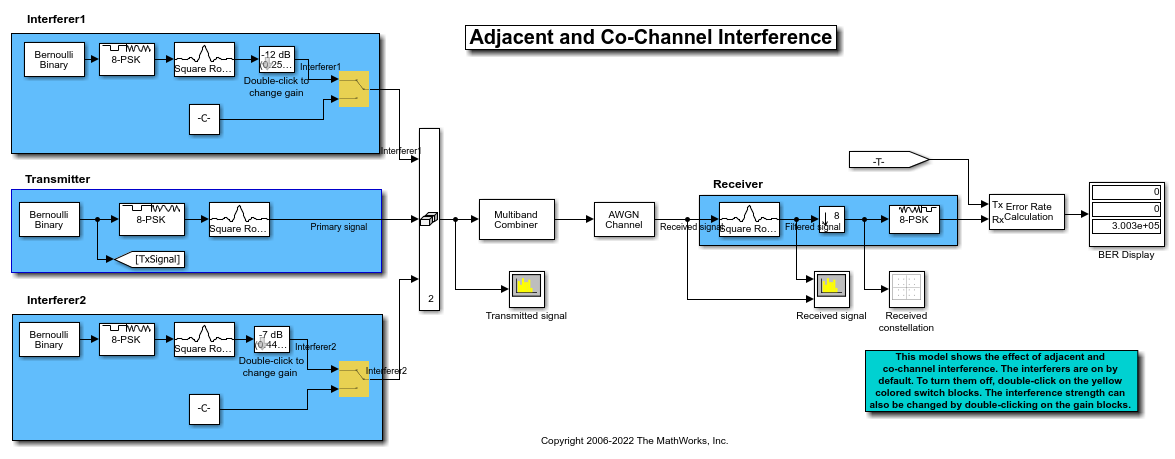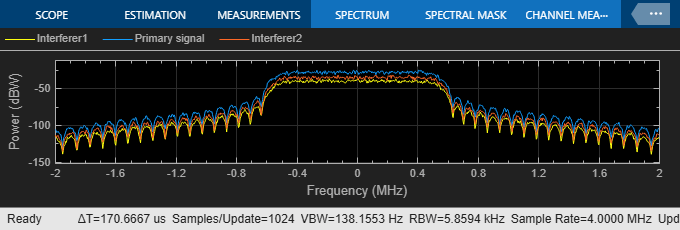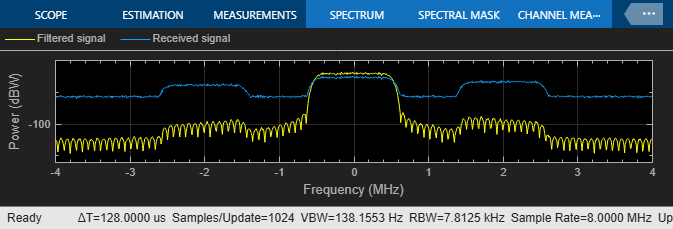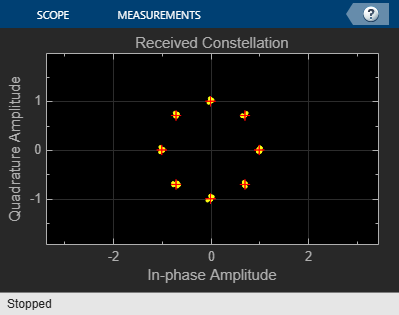Main Content

# Adjacent and Co-Channel Interference

This model shows the effects of adjacent and co-channel interference on a PSK modulated signal. The model includes two interferers, Interferer 1 and Interferer 2. The model enables you to modify the frequency offset and the power gain of each interferer, and view the effects on a spectrum plot.

### Exploring the Example

The communication system in this example includes these components:

• A transmitter, which creates a PSK modulated signal and applies a square root raised cosine filter. The result is the original signal to which interference is added.

• Two interferers, Interferer1 and Interferer2, each of which is similar to the transmitted signal but has a modifiable frequency offset and power gain. A sum block in the model adds the two interfering signals to the original signal. By default, both interferers are active; however, you can deactivate one or both interferers, as described in Experimenting with the Example below.

• An `AWGN channel`.

• A receiver, which filters, downsamples, and demodulates the received signal.### Results and Displays

When you run the simulation, the block labeled BER Display shows the bit error rate for the original signal. The `Display` block shows a three-element vector containing the calculated bit error rate (BER), the number of errors observed, and the number of bits processed.

Scope blocks in the model display these quantities:

• The spectra of the original and interfering signals and the noisy transmitted signal• The spectrum of the received signal• A scatter plot of the received signal constellation### Experimenting with the Example

To deactivate an interferer, double-click the switch block that corresponds to that interferer. In the "Transmitted signal" spectrum analyzer, notice the effect of omitting the interfering signal.

To decrease the frequency offset of an interfering signal, double-click the Slider gain block that corresponds to that interferer and move the slider. As you decrease the offset, the "Transmitted signal" spectrum analyzer shows the interfering signal slowly moving from the adjacent channel into the frequency band of the original signal and eventually causing co-channel interference. The BER values slowly deteriorate as the offset decreases, because the 8-PSK constellation points become difficult to demodulate.

To change the power gain of an interfering signal, double-click the dB Gain block and change the Gain parameter. Observe the effect on the "Transmitted signal" spectrum analyzer. If you decrease the negative dB gain, the BER worsens, especially in the presence of co-channel interference.

## Support

#### Bridging Wireless Communications Design and Testing with MATLAB

Download white paper